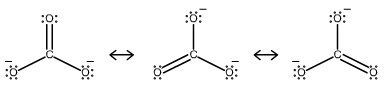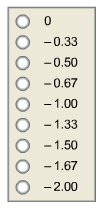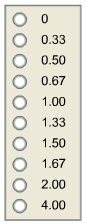# Problem: Consider the resonance structures for the carbonate ion. How much negative charge is on each oxygen of the carbonate ion? What is the bond order of each carbon-oxygen bond in the carbonate ion?

###### FREE Expert Solution

Analyze each structure and determine the charges in each atom. The sum of the charges will be the net charge of the molecule

For the bond order, identify the bonds in the structure.

Step 1. Analyze the resonance structures

• Since the charges of the atoms are already given, we can notice that two of O atoms have a charge of -1 where C and O are neutral.
90% (262 ratings)###### Problem Details

Consider the resonance structures for the carbonate ion.How much negative charge is on each oxygen of the carbonate ion?What is the bond order of each carbon-oxygen bond in the carbonate ion?